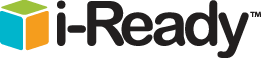•Virtual NerdUSA Test Prep### Basic Math Resources• AAA Math has numerous interactive arithmetic lessons. Topics covered include addition, counting, comparing, decimals, equations, estimations, graphs, fraction, multiplication, and more. You can also sort topics by grade level, starting in kindergarten and ending with eighth grade.
• Aplus Math caters to grades K-12 so you will find a wide variety of math topics here. The site has flashcards, games, homework help, and practice work sheets.
• Math Reference Tables covers general math, algebra, geometry, trigonometry, and above. Its general section includes number notation, addition, multiplication, fractions, and units and measurement. This site can also be translated into Spanish!
• Elementary Mathematics is organized by grade level (K-5). Each grade has different math activities specifically targeted to that age group. Grade 1, for instance, includes information on addition, subtraction, estimating sums, fractions, and much more. Grade 5, on the other hand, has info on measurements, graphs, angles, and dispersion.
• KidZone Math offers helpful math resources in fun, kid-appropriate formats. A range of free, printable worksheets is available, organized by grade level, activity type, and theme, allowing kids to find a worksheet that suits them best. In addition, this site provides timed quizzes on addition, subtraction, and multiplication.
• Math Arcade, has a plethora of games for kids kindergarten to eighth grade to practice their math skills. The site includes addition, subtraction, fractions, division, and much more.
• Math is Fun!, as evident from its name, attempts to present math to kids as an enjoyable, interactive subject. Simple explanations are supplemented by practice problems in the following topics: numbers, algebra, geometry, data (mean, median, mode, surveys, tables), and measurements. Also included are puzzles and quizzes, as well as an elaborate illustrated dictionary of basic math terms.
• Multiplication.com focuses, as one may expect, exclusively on multiplication. The site features games, self-correcting timed quizzes, resources, and instructional videos. This is a great site for any child struggling with his times tables.

BASIC MATH RESOURCES TAKEN FROM:

http://www.studentguide.org/a-complete-list-of-online-math-resources/